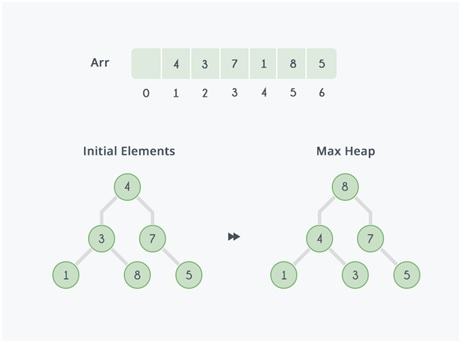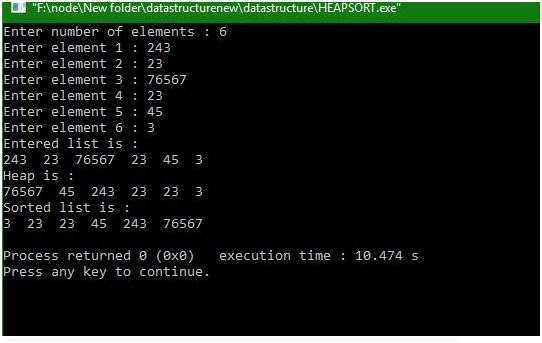# What is heap sort and how it works?

In this article, we will look up on Heap sort, how it works and will try to understand its sorting process through an example.
Submitted by Manu Jemini, on January 18, 2018When we say sorting it means serializing a collection of data inside any the collections like arrays, linked list etc. There is one good reason for implementing sorting on any collection is that it makes the searching very fast as compared to the collection which is unsorted. Now, this is not true for every test case but on an average, it is so.

So what is Heap sort? Well, heaps are of two type max heap and min heap. The Basic and important rule to follow is that in a max heap the parent node should be greater than that of its child.

In the example given above the node 8 is greater than its parent, so we move it up till every node is satisfying the rule.

Similarly, in Min-heap the parent node should be smaller than its child.

Every heap can be represented by a tree; this tree can be an array just like in the example. The First element of the array is the root of the tree. Let’s say its i, so every 2*ith + 1 is the index of the left child of the ith node and 2*ith + 2 is the index of the right child of the ith element.

## C code for heap sort

```# include <stdio.h>

int arr,n;

void insert(int num,int loc)
{	int par;
while(loc>0)
{
par=(loc-1)/2;
if(num<=arr[par])
{
arr[loc]=num;
return;
}
arr[loc]=arr[par];
loc=par;
}/*End of while*/
arr=num;
}/*End of insert()*/

void create_heap()
{
int i;
for(i=0;i<n;i++)
insert(arr[i],i);
}/*End of create_heap()*/

void del_root(int last)
{
int left,right,i,temp;
i=0; /*Since every time we have to replace root with last*/
/*Exchange last element with the root */
temp=arr[i];
arr[i]=arr[last];
arr[last]=temp;

left=2*i+1; /*left child of root*/
right=2*i+2;/*right child of root*/
while( right < last)
{
if( arr[i]>=arr[left] && arr[i]>=arr[right] )
return;
if( arr[right]<=arr[left] )
{temp=arr[i];
arr[i]=arr[left];
arr[left]=temp;
i=left;	}
else
{
temp=arr[i];
arr[i]=arr[right];
arr[right]=temp;
i=right;
}
left=2*i+1;
right=2*i+2;
}/*End of while*/
if( left==last-1 && arr[i]<arr[left] )/*right==last*/
{temp=arr[i];
arr[i]=arr[left];
arr[left]=temp;
}
}/*End of del_root*/

void heap_sort()
{
int last;
for(last=n-1; last>0; last--)
del_root(last);
}/*End of heap_sort*/

void display()
{       int i;
for(i=0;i<n;i++)
printf("%d  ",arr[i]);
printf("\n");
}/*End of display()*/

main()
{
int i;
printf("Enter number of elements : ");
scanf("%d",&n);
for(i=0;i<n;i++)
{
printf("Enter element %d : ",i+1);
scanf("%d",&arr[i]);

}

printf("Entered list is :\n");
display();
create_heap();
printf("Heap is :\n");
display();
heap_sort();
printf("Sorted list is :\n");
display();
}/*End of main()*/
```

Output